### Home > A2C > Chapter 4 > Lesson 4.1.2 > Problem4-29

4-29.
1. This is a Checkpoint for writing the equation of a line given two points. Homework Help ✎Write the linear equation for each line described in parts (a) and (b) below.

1. The line that goes through the points (−1, 4) and (2, 1).

2. The line that goes through the points (−8, 18) and (4, 9).

3. The line that passes through the points (−1, −2) and (11, 2).

4. Check your answers by referring to the Checkpoint 6 materials located at the back of your book.

If you needed help to solve these problems correctly, then you need more practice writing the equation of a line through two given points. Review the Checkpoint 6 materials and try the practice problems. Also, consider getting help outside of class time. From this point on, you will be expected to find equations of lines such as these quickly and easily.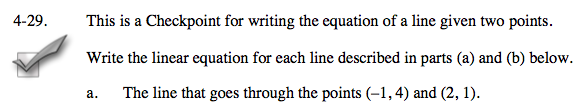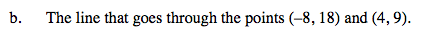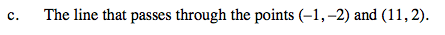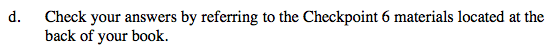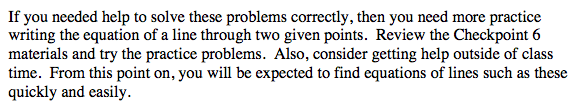Answers and extra practice are located in the back of your printed textbook or in the Reference Tab of your eBook.
If you have an eBook for A2C, login and then click the following link: Checkpoint 6 Answers and Practice